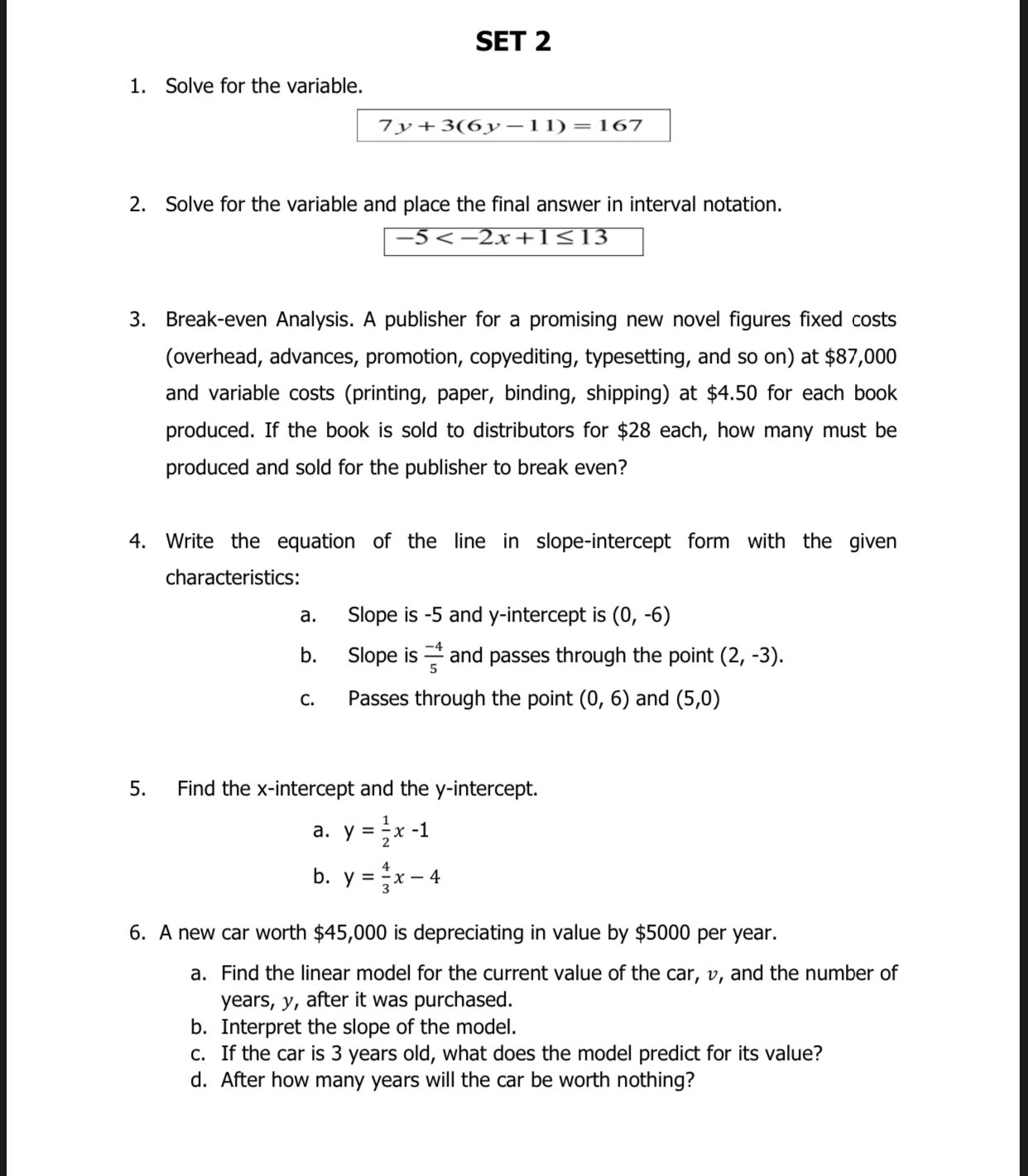### ¿Todavía tienes preguntas de matemáticas?

Pregunte a nuestros tutores expertos
Algebra
Pregunta1. Solve for the variable. $$7 y + 3 ( 6 y - 11 ) = 167$$.

2. Solve for the variable and place the final answer in interval notation. $$- 5 < - 2 x + 1 \leq 13$$.

3. Break-even Analysis. A publisher for a promising new novel figures fixed costs (overhead, advances, promotion, copyediting, typesetting, and so on) at $$\ 87,000$$ and variable costs (printing, paper, binding, shipping) at $$\ 4.50$$ for each book produced. If the book is sold to distributors for $$\ 28$$ each, how many must be produced and sold for the publisher to break even?

4. Write the equation of the line in slope-intercept form with the given characteristics:

a. Slope is $$- 5$$ and $$y$$ -intercept is $$( 0 , - 6 )$$

b. Slope is $$\frac { - 4 } { 5 }$$ and passes through the point $$( 2 , - 3 )$$ .

c. Passes through the point $$( 0,6 )$$ and $$( 5,0 )$$

5. Find the $$x$$ -intercept and the $$y$$ -intercept.

a. $$y = \frac { 1 } { 2 } x - 1$$

b. $$y = \frac { 4 } { 3 } x - 4$$

6. A new car worth $$\ 45,000$$ is depreciating in value by $$\ 5000$$ per year.

a. Find the linear model for the current value of the car, $$v$$ , and the number of years, $$y$$ , after it was purchased.

b. Interpret the slope of the model.

c. If the car is $$3$$ years old, what does the model predict for its value?

d. After how many years will the car be worth nothing?

1. y=8

2. x<3

5. a. -1

b. -4

6. a. v=45000-5000y

b. slope=-5000

c. \$30000

d. 9 years

Solución
View full explanation on CameraMath App.